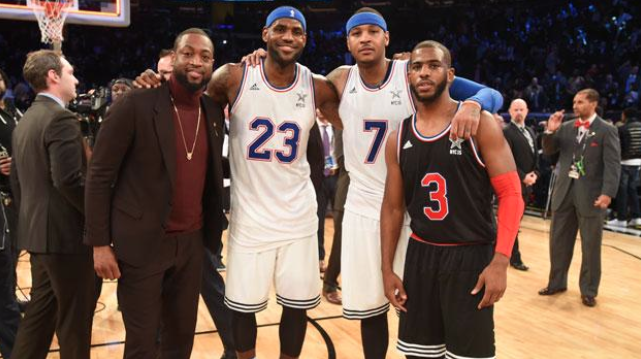## Tuesday, March 31, 2015

### 33 | LeBron & His 3 NBA Friends23+7+3 = 33
Today we're told LeBron has three friends in the NBA, the photo is of them above.  Notice that "Wade" is not in uniform.
• Wade = 23+1+4+5 = 33
• 23+7+3 = 33
• LeBron = 12+5+2+18+15+14 = 66
• Thirty-Three = 66 (Pythagorean Gematria)
• Cleveland = 33
Are you getting it truth seeker?

The NBA is a '33' league, from Bird to Magic to the NBA Finals and beyond.
• Bird = 2+9+18+4 = 33 (Wore 33)(Massachusetts = 33)
• Magic = 13+1+7+9+3 = 33 (Earvin = 33)(Wore 33 at Michigan State)
• NBA Finals = 33 (Pythagorean Gematria)
• Patrick = 33
• Riley = 33
• Riley has the "TM" on 3-Peat
• TM = 20+13 = 33
• So much more...
• Michael Jordan = 113
• Kobe Bryant = 113